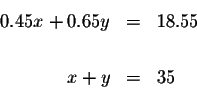#SYSTEMS OF EQUATIONSA system of equations is a collection of two or more equations with a same set of unknowns. In solving a system of equations, we try to find values for each of the unknowns that will satisfy every equation in the system.

The equations in the system can be linear or non-linear. This tutorial reviews systems of linear equations.

The problem can be expressed in narrative form or the problem can be expressed in algebraic form.

Example of a narrative statement of a system of the equations:

The air-mail rate for letters to Europe is 45 cents per half-ounce and to Africa as 65 cents per half-ounce. If Shirley paid \$18.55 to send 35 half-ounce letters abroad, how many did she send to Africa?

Example of an algebraic statement of the same system of the equations:A system of linear equations can be solved four different ways:

Substitution

Gaussian Elimination

Matrices

Graphing

In this review, we are going to show you how to convert a narrative statement of the problem to an equivalent algebraic of the problem. In other words, we are going to show you how to translate word problems in to algebraic equations.

We are also going to show you how to work and check each example by each of the four methods. The review has several levels. If you find that you easily understand the example, you can go on to new material. If you are having trouble with an example, you can work many examples of the same type and difficulty. We have imbedded the tutorial with many self-tests. At any time during your review, you may test your knowledge of the material covered.

This tutorial will cover systems in two variables, three variables, and four variables.

Click on the system you wish to review:

A system of linear equations in two variables.

A system of linear equations in three variables.

This site was built to accommodate the needs of students of all ages and disciplines. the topics and problems are what students request. We ask you to help in the editing so that future viewers will access a cleaner site. If you feel that some of the material in this section is ambiguous or need more clarification, please let us know by email at sosmath.com.[Two-Variable Systems] [Three-Variable Systems]
[Algebra] [Geometry] [Trigonometry ]S.O.S MATH: Home Page

Do you need more help? Please post your question on our S.O.S. Mathematics CyberBoard.Author: Nancy Marcus